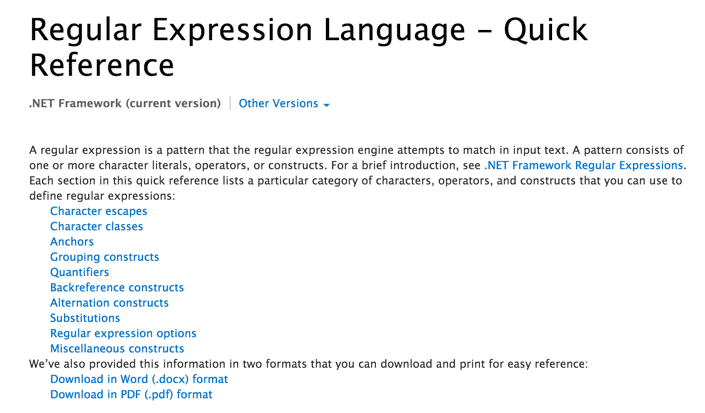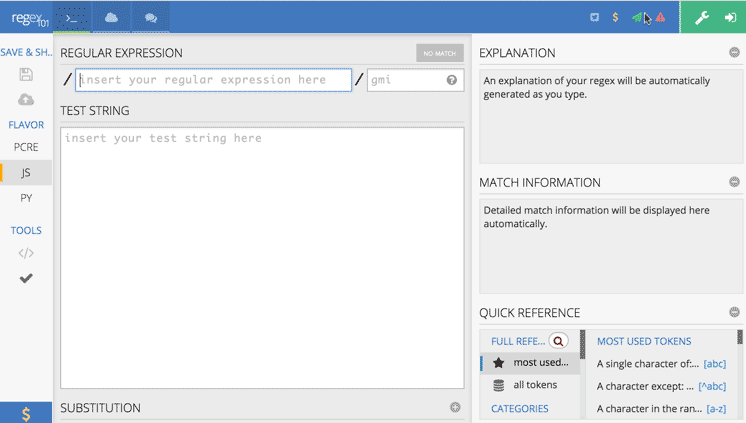# 必须知道的20个正则表达式

``````
var reg = /^(\\d{1,4})(-|\\/)(\\d{1,2})\\2(\\d{1,2})\$/;
var r = fieldValue.match(reg);
if(r==null)alert('Date format error!'); ``````

1 . 校验密码强度

``````
^(?=.*\\d)(?=.*[a-z])(?=.*[A-Z]).{8,10}\$ ``````

2. 校验中文

``````
^[\\u4e00-\\u9fa5]{0,}\$ ``````

3. 由数字、26个英文字母或下划线组成的字符串

``````
^\\w+\$ ``````

4. 校验E-Mail 地址

``````
[\\w!#\$%&'*+/=?^_`{|}~-]+(?:\\.[\\w!#\$%&'*+/=?^_`{|}~-]+)*@(?:[\\w](?:[\\w-]*[\\w])?\\.)+[\\w](?:[\\w-]*[\\w])? ``````

5. 校验身份证号码

15位：

``````
^[1-9]\\d{7}((0\\d)|(1[0-2]))(([0|1|2]\\d)|3[0-1])\\d{3}\$ ``````

18位：

``````
^[1-9]\\d{5}[1-9]\\d{3}((0\\d)|(1[0-2]))(([0|1|2]\\d)|3[0-1])\\d{3}([0-9]|X)\$ ``````

6. 校验日期

“yyyy-mm-dd“ 格式的日期校验，已考虑平闰年。

``````
^(?:(?!0000)[0-9]{4}-(?:(?:0[1-9]|1[0-2])-(?:0[1-9]|1[0-9]|2[0-8])|(?:0[13-9]|1[0-2])-(?:29|30)|(?:0|1)-31)|(?:[0-9]{2}(?:0||)|(?:0||)00)-02-29)\$``````

7. 校验金额

``````
^[0-9]+(.[0-9]{2})?\$ ``````

8. 校验手机号

``````
^(13[0-9]|14[5|7]|15[0|1|2|3|5|6|7|8|9]|18[0|1|2|3|5|6|7|8|9])\\d{8}\$ ``````

9. 判断IE的版本

IE目前还没被完全取代，很多页面还是需要做版本兼容，下面是IE版本检查的表达式。

``````
^.*MSIE [5-8](?:\\.[0-9]+)?(?!.*Trident\\/[5-9]\\.0).*\$ ``````

10. 校验IP-v4地址

IP4 正则语句。

\\b(?:(?:25[0-5]|2[0-4][0-9]|?[0-9][0-9]?)\\.){3}(?:25[0-5]|2[0-4][0-9]|?[0-9][0-9]?)\\b

11. 校验IP-v6地址

IP6 正则语句。

``````
(([0-9a-fA-F]{1,4}:){7,7}[0-9a-fA-F]{1,4}|([0-9a-fA-F]{1,4}:){1,7}:|([0-9a-fA-F]{1,4}:){1,6}:[0-9a-fA-F]{1,4}|([0-9a-fA-F]{1,4}:){1,5}(:[0-9a-fA-F]{1,4}){1,2}|([0-9a-fA-F]{1,4}:){1,4}(:[0-9a-fA-F]{1,4}){1,3}|([0-9a-fA-F]{1,4}:){1,3}(:[0-9a-fA-F]{1,4}){1,4}|([0-9a-fA-F]{1,4}:){1,2}(:[0-9a-fA-F]{1,4}){1,5}|[0-9a-fA-F]{1,4}:((:[0-9a-fA-F]{1,4}){1,6})|:((:[0-9a-fA-F]{1,4}){1,7}|:)|fe80:(:[0-9a-fA-F]{0,4}){0,4}%[0-9a-zA-Z]{1,}|::(ffff(:0{1,4}){0,1}:){0,1}((25[0-5]|(2[0-4]|1{0,1}[0-9]){0,1}[0-9])\\.){3,3}(25[0-5]|(2[0-4]|1{0,1}[0-9]){0,1}[0-9])|([0-9a-fA-F]{1,4}:){1,4}:((25[0-5]|(2[0-4]|1{0,1}[0-9]){0,1}[0-9])\\.){3,3}(25[0-5]|(2[0-4]|1{0,1}[0-9]){0,1}[0-9])) ``````

12. 检查URL的前缀

``````
if (!s.match(/^[a-zA-Z]+:\\/\\//))
{
s = 'http://' + s;
}``````

13. 提取URL链接

``````
^(f|ht){1}(tp|tps):\\/\\/([\\w-]+\\.)+[\\w-]+(\\/[\\w- ./?%&=]*)? ``````

14. 文件路径及扩展名校验

``````
^([a-zA-Z]\\:|\\\\)\\\\([^\\\\]+\\\\)*[^\\/:*?"<>|]+\\.txt(l)?\$ ``````

15. 提取Color Hex  Codes

``````
\\#([a-fA-F]|[0-9]){3,6} ``````

16. 提取网页图片

``````
\\< *[img][^\\>]*[src] *= *[\\"\\']{0,1}([^\\"\\'\\ >]*) ``````

17. 提取页面超链接

``````
(<;a\\s*(?!.*\\brel=)[^>;]*)(href="https?://)((?!(?:(?:www\\.)?'.implode('|(?:www\\.)?', \$follow_list).'))[^"]+)"((?!.*\\brel=)[^>;]*)(?:[^>;]*)> ``````

18. 精炼CSS

``````
^\\s*[a-zA-Z\\-]+\\s*[:]{1}\\s[a-zA-Z0-9\\s.#]+[;]{1} ``````

19. 抽取注释

<!--(.*?)-->

20. 匹配HTML标签

``````
</?\\w+((\\s+\\w+(\\s*=\\s*(?:".*?"|'.*?'|[\\^'">\\s]+))?)+\\s*|\\s*)/?> ``````regex101是一个非常不错的正则表达式在线测试工具，你可以直接在线测试你的正则表达式哦。### 正则表达式-人气榜

QQ群:153638182  投稿邮箱: 578640261@qq.com  皖ICP备-14005058